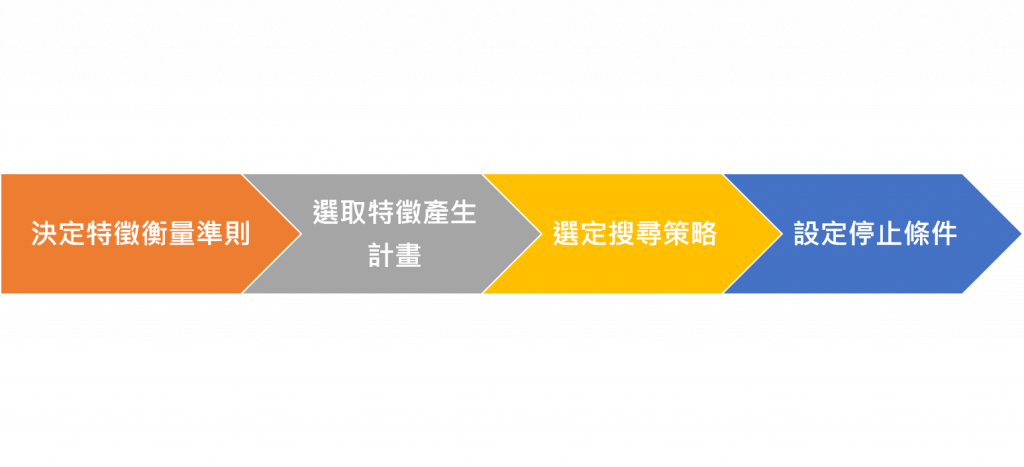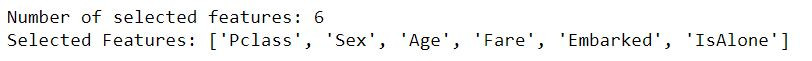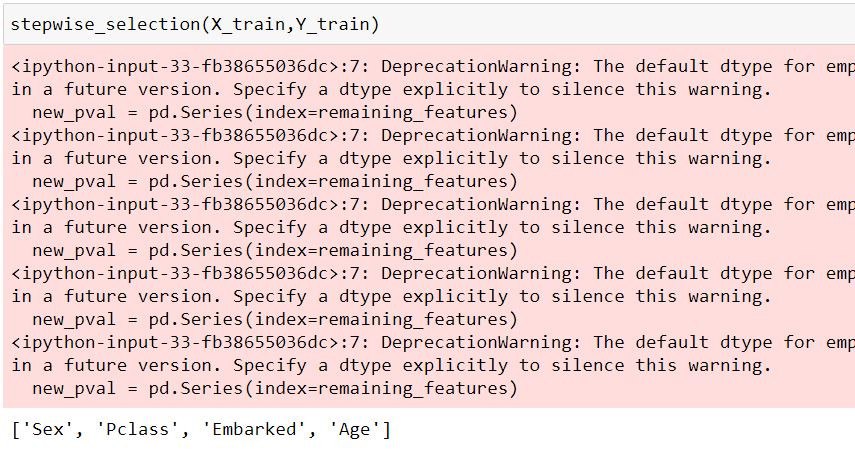#DAY 12
0
AI & Data

## 一、特徵選取(Feature Selection)

### 3. Avoiding Curse of Dimensionality (避免維度災難)## 二、過濾法(Filter)

### 常數特徵(Constant Feature)：一個特徵下的值完全一樣，沒有變化。

``````from sklearn.feature_selection import VarianceThreshold
constant = VarianceThreshold(threshold=0)
constant.fit(x_train) #fit我們的資料集
# 得到常數特徵的欄位
constant_columns = [column for column in x_train.columns
if column not in
x_train.columns[constant.get_support()]]

print(constant_columns)
``````

### 半常數特徵(Quasi-Constant Feature)：特徵裡大部分都是同一個數值。

``````
# 設定門檻，要刪除幾%的資料
threshold = 0.95
quasi_constant_feature = [] #用來記錄的list

for i in x_train.columns: #每個特徵依序看

# 計算比率
predominant = (x_train[i].value_counts() /
np.float(len(x_train))).sort_values(ascending=False).values

# 假如大於門檻 加入 list
if predominant >= threshold:
quasi_constant_feature.append(i)

print(quasi_constant_feature)
``````

### 重複特徵(Duplicated Feature)：資料集有兩個以上完全一樣的特徵。

``````# 轉置特徵矩陣
train_features_T = x_train.T

#找出重複的欄位
duplicated_columns = train_features_T[train_features_T.duplicated()].index.values

print(duplicated_columns )
``````

### 挑選方法

● SelectKBest：選取 K 個最好的特徵，k 為參數，代表選擇的特徵數。

● SelectPercentile：選取多少百分比的特徵，percentile 為參數，代表百分比，用 10 代表 10%。

### 卡方檢定(Chi2)-用於離散型目標變數

``````from sklearn.feature_selection import SelectKBest
from sklearn.feature_selection import chi2
#離散型資料要先轉成數值

x=_train_df[['Pclass', 'Sex', 'Age', 'Fare', 'Embarked', 'IsAlone','Cabin']]
y=data['Survived']

x_new = SelectKBest(chi2, k=2).fit_transform(x, y) #挑選2個最好的特徵
display(x_new)
``````

### f_regression-用於連續型目標變數

``````from sklearn.feature_selection import SelectKBest
from sklearn.feature_selection import f_regression

x=data[['前一天價格','前一季價格', '前一年價格','同性質股票價格']]
y=data['Price']

x_new = SelectPercentile(chi2, percentile=50).fit_transform(x, y) #取前50%的特徵

display(x_new)
``````

## 三、包裝法(Wrapper)

RFE是根據問題為離散或連續，利用機器學習的模型進行挑選，為一貪婪優化演算法,目的在找尋最佳的特徵子集。

### RFE

``````#RFE
from sklearn.feature_selection import RFE
rf = RandomForestClassifier()
rfe = RFE(rf, 6) #篩選6個特徵
rfe.fit(X_train, Y_train)

print(f"Number of selected features: {rfe.n_features_}\n\
Selected Features:", [feature for feature, rank in zip(X_train.columns.values, rfe.ranking_) if rank==1]) #列出挑選的6個變數
``````### 程式碼實現

``````import statsmodels.api as sm
import pandas as pd
#Stepwise
def stepwise_selection(data, target,SL_in=0.05,SL_out = 0.05):
initial_features = data.columns.tolist()
best_features = []
while (len(initial_features)>0):
remaining_features = list(set(initial_features)-set(best_features))
new_pval = pd.Series(index=remaining_features)
for new_column in remaining_features:
new_pval[new_column] = model.pvalues[new_column]
min_p_value = new_pval.min()
if(min_p_value<SL_in):
best_features.append(new_pval.idxmin())
while(len(best_features)>0):
p_values = sm.OLS(target, best_features_with_constant).fit().pvalues[1:]
max_p_value = p_values.max()
if(max_p_value >= SL_out):
excluded_feature = p_values.idxmax()
best_features.remove(excluded_feature)
else:
break
else:
break
return best_features
``````